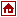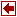## Nonlinear Programming Packages

 OPTIMA Library Author Dr. M. C. Bartholomew-Biggs Numerical Optimisation Center Hatfield, Hertfordshire AL10 9AB United Kingdom Language FORTRAN 77 Algorithm Input Format Modeling Languages link Commercial Status Platform Remarks The contents of the library is as follows: OPVM --- Unconstrained optimization, unstructured objective function; suitable for small problems. OPVMB --- Optimization subject to simple bounds. OPLS --- Unconstrained nonlinear least squares. OPNL --- Nonlinear equations, by minimizing sum of squares of the residuals. OPCG --- Nonlinear conjugate gradient method. OPODEU --- Unconstrained optimization problems by tracing the solution curve of a system of ODEs (homotopy method). OPTNHP --- Unconstrained optimization using the truncated Newton method; no Hessian storage or calculation required. OPRQP --- Sequential quadratic programming, but superseded by the OPXRQP routine. OPXRQP --- A more efficient implementation of sequential quadratic programming; uses the EQP variant. OPSQP --- Another implementation of sequential quadratic programming, but uses the IQP variant, which gives rise to inequality-constrained subproblems. OPALQP --- Similar to OPSQP, but uses an augmented Lagrangian line search function. OPSMT --- Nonlinear programming, using a SUMT technique. OPIPF --- Sequential minimization of a sequence of augmented Lagrangians. OPODEC --- Homotopy method: traces the solution curve of a system of ODEs. OPSEN --- Tests the sensitivity of the objective function around the optimal point of an unconstrained problem. OPSEC --- Like OPSEN, but for the solution of a constrained problem. References OPTIMA Manual, Numerical Optimisation Center, University of Hertfordshire, UK, July 1989.